## Screenshots

•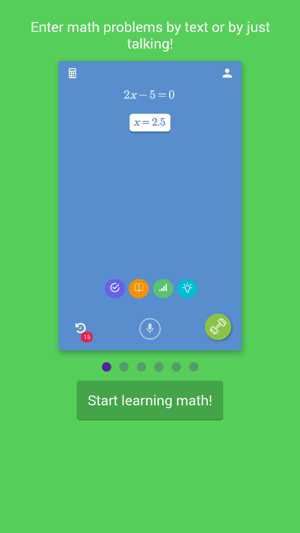•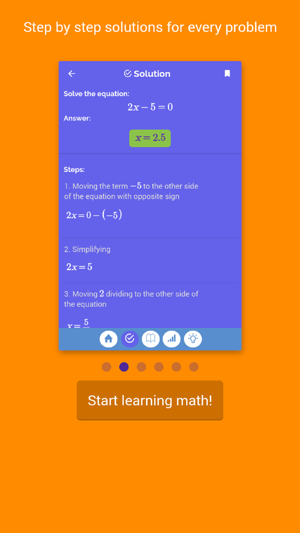•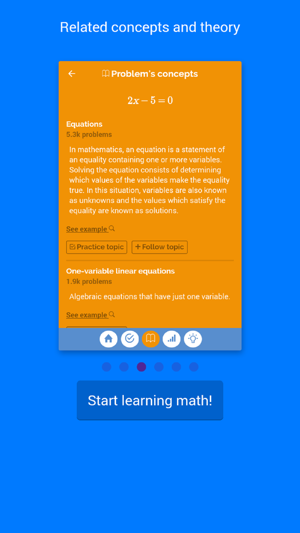•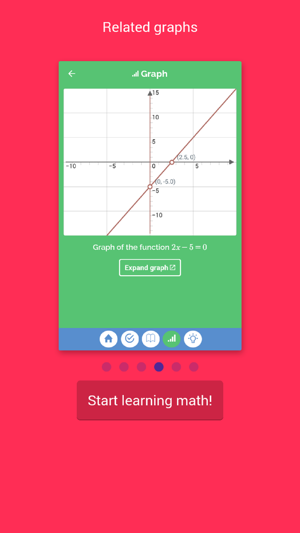•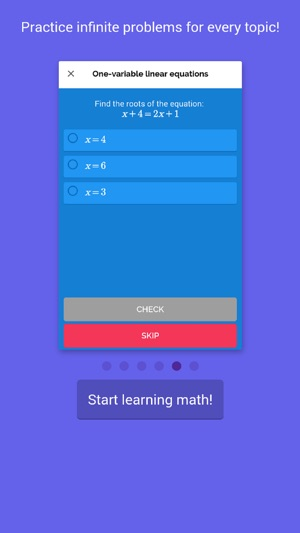## Description

OneSecondSolver is a math tutor available anywhere anytime!! Just type in the equation you want to solve and instantly get human-like detailed explanations.

OneSecondSolver currently supports:
• Arithmetic
• Pre-Algebra
• Algebra
• Linear and quadratic equations
• Linear and quadratic inequations
• Evaluating Functions, like f(0)
• Polynomial Long Division
• Proving Trigonometric Identities

Special Products:
• Distributive Law
• Difference of squares
• Sum and difference of two cubes
• Completing the square

Calculus:
• Limits by factoring
• Limits by rationalization
• Limits by double rationalization
• Limits by L'Hospital's Rule

• Derivatives
• Derivatives by definition

• Integrals
• Definite Integrals
• Trigonometric Integrals
• Integrals by substitution
• Integrals by parts
• Integrals by partial fractions
• Integrals by trigonometric substitution
• Integrals using Taylor Series

• Differential Equations

Upcoming features:
• Improper Integrals
• Laplace Transform
• Fourier Transform

## What’s New

Version 3.2.0

* UX improved
* now, we have videos !

## Information

Provider
Juan Giron
Size
25.4 MB
Category
Education
Compatibility

Requires iOS 10.0 or later. Compatible with iPhone, iPad and iPod touch.

Languages

English

Age Rating
Rated 4+
•